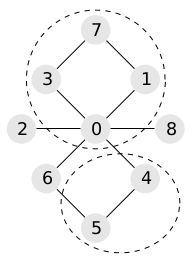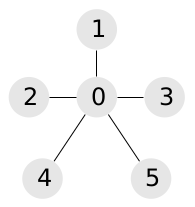# #535. 【IOI2019】景点划分

Fatima 打算在三天之内参观完所有这些景点。她已经决定要在第一天参观 $a$ 处景点，第二天参观 $b$ 处景点，第三天参观 $c$ 处景点。因此，她要把这 $n$ 处景点划分为三个集合 $A,B$ 和 $C$，其规模分别为 $a,b$ 和 $c$ 。每处景点恰好属于其中一个集合，因此有 $a+b+c=n$ 。

Fatima 想要找到这样的集合划分 $A,B$ 和 $C$，使得这三个集合中的至少两个是连通的。一个景点集合 $S$ 被称为是连通的，如果能够经由这些道路在 $S$ 中的任意两处景点之间往来，且不需要经过不在 $S$ 中的景点。如果满足上述要求，则景点的一个划分 $A,B$ 和 $C$ 被称为是合法的。

### 样例一

#### input

9 10
4 2 3
0 1
0 2
0 3
0 4
0 6
0 8
1 7
3 7
4 5
5 6

#### output

1 1 3 1 2 2 3 1 3

#### explanation### 样例二

#### input

6 5
2 2 2
0 1
0 2
0 3
0 4
0 5

#### output

0 0 0 0 0 0

#### explanation### 数据范围

$1$每处景点至多可做两条道路的端点7
$2$$a=111 3$$m=n-1$22
$4$$n \le 2500,m \le 5000$24
$5$无特殊约定36